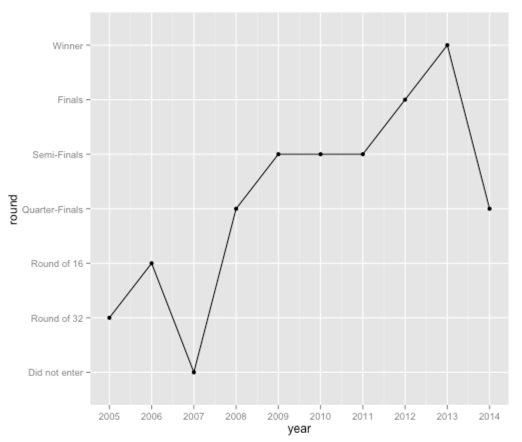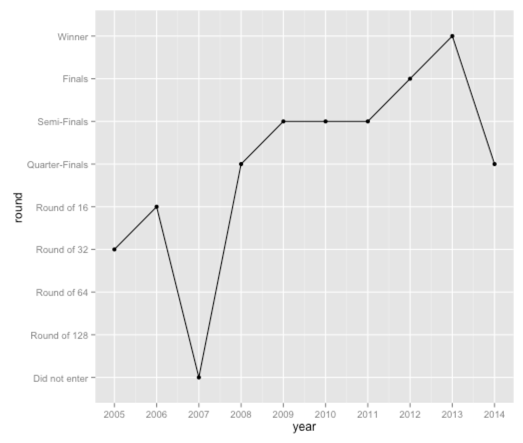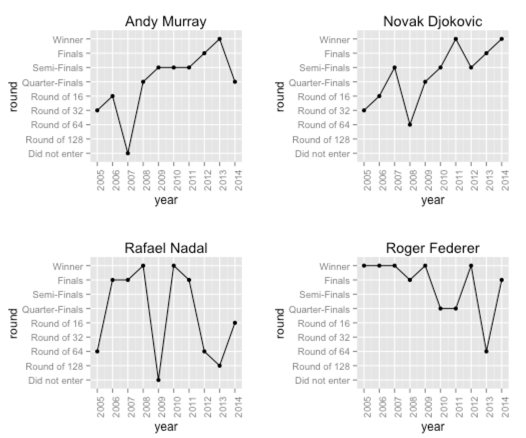· r-2

# R: ggplot - Show discrete scale even with no value

As I mentioned in a previous blog post, I’ve been scraping data for the Wimbledon tennis tournament, and having got the data for the last ten years I wrote a query using dplyr to find out how players did each year over that period.

I ended up with the following functions to filter my data frame of all the matches:

``````round_reached = function(player, main_matches) {
furthest_match = main_matches %>%
filter(winner == player | loser == player) %>%
arrange(desc(round)) %>%

return(ifelse(furthest_match\$winner == player, "Winner", as.character(furthest_match\$round)))
}

player_performance = function(name, matches) {
player = data.frame()
for(y in 2005:2014) {
round = round_reached(name, filter(matches, year == y))
if(length(round) == 1) {
player = rbind(player, data.frame(year = y, round = round))
} else {
player = rbind(player, data.frame(year = y, round = "Did not enter"))
}
}
return(player)
}``````

When we call that function we see the following output:

``````> player_performance("Andy Murray", main_matches)
year          round
1  2005    Round of 32
2  2006    Round of 16
3  2007  Did not enter
4  2008 Quarter-Finals
5  2009    Semi-Finals
6  2010    Semi-Finals
7  2011    Semi-Finals
8  2012         Finals
9  2013         Winner
10 2014 Quarter-Finals``````

I wanted to create a chart showing Murray’s progress over the years with the round reached on the y axis and the year on the x axis. In order to do this I had to make sure the 'round' column was being treated as a factor variable:

``````df = player_performance("Andy Murray", main_matches)

rounds = c("Did not enter", "Round of 128", "Round of 64", "Round of 32", "Round of 16", "Quarter-Finals", "Semi-Finals", "Finals", "Winner")
df\$round = factor(df\$round, levels =  rounds)

> df\$round
 Round of 32    Round of 16    Did not enter  Quarter-Finals Semi-Finals    Semi-Finals    Semi-Finals
 Finals         Winner         Quarter-Finals
Levels: Did not enter Round of 128 Round of 64 Round of 32 Round of 16 Quarter-Finals Semi-Finals Finals Winner``````

Now that we’ve got that we can plot his progress:

``````ggplot(aes(x = year, y = round, group=1), data = df) +
geom_point() +
geom_line() +
scale_x_continuous(breaks=df\$year) +
scale_y_discrete(breaks = rounds)``````This is a good start but we’ve lost the rounds which don’t have a corresponding entry on the x axis. I’d like to keep them so it’s easier to compare the performance of different players.

It turns out that all we need to do is pass 'drop = FALSE' to scale_y_discrete and it will work exactly as we want:

``````ggplot(aes(x = year, y = round, group=1), data = df) +
geom_point() +
geom_line() +
scale_x_continuous(breaks=df\$year) +
scale_y_discrete(breaks = rounds, drop = FALSE)``````Neat. Now let’s have a look at the performances of some of the other top players:

``````draw_chart = function(player, main_matches){
df = player_performance(player, main_matches)
df\$round = factor(df\$round, levels =  rounds)

ggplot(aes(x = year, y = round, group=1), data = df) +
geom_point() +
geom_line() +
scale_x_continuous(breaks=df\$year) +
scale_y_discrete(breaks = rounds, drop=FALSE) +
ggtitle(player) +
theme(axis.text.x=element_text(angle=90, hjust=1))
}

a = draw_chart("Andy Murray", main_matches)
b = draw_chart("Novak Djokovic", main_matches)